# REASONING (Test 3)

Tag: reasoning
Q.1
What comes next?A.B.C.D.Explaination / Solution:

The inner hexagon is being dismantled one side at a time working anti clockwise, while the outer hexagon is being constructed one side at a time working clockwise.

Workspace
Report
Q.2
A person X has four notes of Rupee 1, 2 , 5 and 10 denomination. The number of different sums of money she can form from them is
A. 16
B. 15
C. 12
D. 8
Explaination / Solution:
No Explaination.

Workspace
Report
Q.3
Running at a speed of 60 km per hour, a train passed through a 1.5 km long tunnel in two minutes. What is the length of the train?
A. 250 m
B. 500 m
C. 1000 m
D. 1500 m
Explaination / Solution:
No Explaination.

Workspace
Report
Q.4
A gardener increased the area of his rectangular garden by increasing its length by 40% and decreasing its width by 20%. The area of the new garden
A. has increased by 20%.
B. has increased by 12%.
C. has increased by 8%.
D. is exactly the same as the old area.
Explaination / Solution:
No Explaination.

Workspace
Report
Q.5

Consider the following matrix:

 3 370 7 2 224 6 1 730 X

What is the number at ‘X’ in the above matrix?

A. 5
B. 8
C. 9
D. 11
Explaination / Solution:
No Explaination.

Workspace
Report
Q.6
Find the number of triangles in the given figure.A. 22
B. 24
C. 26
D. 28
Explaination / Solution:

The figure may be labelled as shown.The simplest triangles are AGH, GFO, LFO, DJK, EKP, PEL and IMN i.e. 7 in number.

The triangles having two components each are GFL, KEL, AMO, NDP, BHN, CMJ, NEJ and HFM i.e. 8 in number.

The triangles having three components each are IOE, IFP, BIF and CEI i.e. 4 in number.

The triangles having four components each are ANE and DMF i.e. 2 in number.

The triangles having five components each are FCK, BGE and ADL i.e. 3 in number.

The triangles having six components each are BPF, COE, DHF and AJE i.e. 4 in number.

Total number of triangles in the figure = 7 + 8 + 4 + 2 + 3 + 4 = 28.

Workspace
Report
Q.7
Find the number of triangles in the given figure.A. 5
B. 6
C. 8
D. 10
Explaination / Solution:

The figure may be labelled as shown.The simplest triangles are AJF, FBG, GCH, HDI and IEJ i.e. 5 in number.

The triangles composed of three components each EBH, AIC, EFC, ADG and BJD i.e. 5 in number.

Thus, there are 5 + 5 = 10 triangles in the figure.

Workspace
Report
Q.8

Find the number of triangles in the given figure.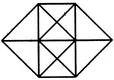A. 20
B. 24
C. 28
D. 32
Explaination / Solution:

The figure may be labelled as shown.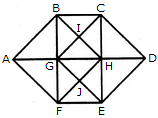The simplest triangles are ABG, BIG, BIC, CIH, GIH, CDH, HED, GHJ, HJE, FEJ, GFJ and AGF i.e. 12 in number.

The triangles composed of two components each are ABF, CDE, GBC, BCH, GHG, BHG, GHF, GHE, HEF and GEF i.e. 10 in number.

The triangles composed of three components each are ABH, AFH, CDG and GDE i.e. 4 in number.

The triangles composed of four components each are BHF and CGE i.e. 2 in number.

Total number of triangles in the figure = 12 + 10 + 4 + 2 = 28.

Workspace
Report
Q.9

Find the number of quadrilaterals in the given figure.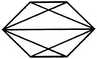A. 6
B. 7
C. 9
D. 11
Explaination / Solution:

The figure may be labelled as shown.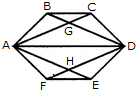The quadrilaterals in the figure are ABCD, ABDE, ABDF, ABDH, CDHA, CDEA, CDFA, DEAG, DEFA, FAGD and AGDH.

The number of quadrilaterals in the figure is 11.

Workspace
Report
Q.10

Count the number of squares in the given figure.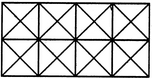A. 11
B. 21
C. 24
D. 26
Explaination / Solution:

The figure may be labelled as shown.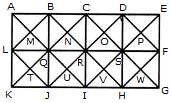The squares composed of two components each are BNQM, CORN, DPSO, MQTL, NRUQ, OSVR, PFWS, QUJT, RVIU and SWHV i.e. 10 in number.

The squares composed of four components each are ABQL, BCRQ, CDSR, DEFS, LQJK, QRIJ, RSHI and SFGH i.e. 8 in number.

The squares composed of eight components each are BRJL, CSIQ and DFHR i.e. 3 in number.

The squares composed of sixteen components each are ACIK, BDHJ and CEGI i.e. 3 in number.

Thus, there are 10 + 8 + 3 + 3 = 24 squares in the figure.

Workspace
Report